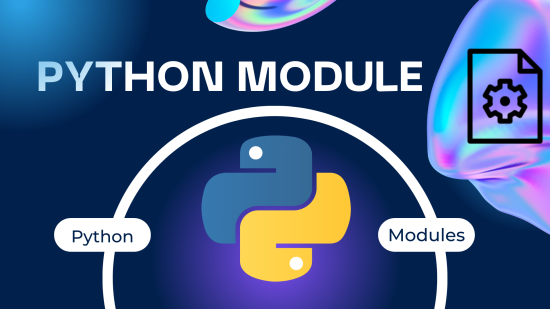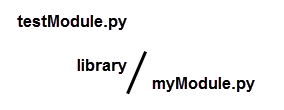# Python Module

Jun 24, 2023## 1. What is a Python module ?

• In Python, a module is a file containing Python definitions, statements, and functions that can be imported and used in other Python programs. It is a way to organize and reuse code by separating it into logical units.
• A Python module can contain variables, functions, and classes that provide specific functionality. By importing a module into a Python program, you can access and use the code defined in that module.
• Python modules are an essential part of the language’s modular programming approach, allowing developers to create reusable and organized code components that can be easily shared and imported into other programs.

## 2. Creating and importing a module

We will try to create our own Python module named myModule:

### 2.2 We introduce a code of some simple functions on the myModule.py file

For example:

```def sum(x , y):
return x + y
def division(x , y):
return x/y```

### 2.3 We then create a python file to test the module

For example testModule.py in the same directory as the file myModule.py (the two files myModule.py and testModule.py can be placed on different directories provided you specify the path of the myModule.py files when it is imported)

### 3.4 On the testModule.py file, type the code:

```# We import the entire module
from myModule import *
# We can now use the module functions:
print ("the sum of 7 and 8 is:", sum (7 , 8))
print ("the division of 12 by 3 is:", division (12 , 3))```

## 3. Partial import of module

To use the existing functions in a module, it is not necessary to import the entire module, but just import the functions you need. For example if we need to use only the sum() function, we just import this function:

```# We import the sum () function of the module
from myModule import sum
# We can now use the sum functions:
print ("the sum of 5 and 3 is:", sum (5 , 3))
# output: the sum of 5 and 3 is: 8```

## 4. Import of an external module

So far, we’ve only seen modules that are in the same directory in the Python file that calls the module, but in general, python modules are stored in other directories and accessible via specific paths. We will deal with a simple example to understand: It is assumed that the file myModule.py is located in a library directory and will therefore be accessible via the path: “/library/myModule.py”:In this case we must specify the path in the import instruction:

```# we specify the module path
from library.myModule import *
# We can now use the module functions:
print ("the sum of 7 and 8 is:", sum (7 , 8))
print ("the division of 12 by 3 is:", division (12 , 3))```

## 5. Import module as alia name

In Python, you can use the import statement to import a module. By default, the module name is used to access its contents. However, if you want to import a module with a different name or alias, you can use the as keyword. This is known as importing a module “as” a different name.

Here’s the syntax for importing a module as a different name:

`import module_name as alias_name`

#### Example

```import math as m

print("The value of PI is : " , m.pi)
# output: The value of PI is :  3.141592653589793```

## 6. List and display elements of a python module

To list and display all elements of a Python module, you can make use of the dir() function. The dir() function returns a list of names in the current module or the names of an object if an object is passed as an argument. Here’s the syntax:

```import module_name

# List the elements in the module
elements = dir(module_name)
print(elements)```

#### Example (list elements of math module)

```import math

# List the elements in the math module
elements = dir(math)
print(elements)
"""
output:
'__spec__', 'acos', 'acosh', 'asin', 'asinh', 'atan',
'atan2', 'atanh', 'ceil', 'comb', 'copysign', 'cos',
'cosh', 'degrees', 'dist', 'e', 'erf', 'erfc', 'exp',
'expm1', 'fabs', 'factorial', 'floor', 'fmod', 'frexp',
'fsum', 'gamma', 'gcd', 'hypot', 'inf', 'isclose',
'isfinite', 'isinf', 'isnan', 'isqrt', 'lcm', 'ldexp',
'lgamma', 'log', 'log10', 'log1p', 'log2', 'modf',
'nan', 'nextafter', 'perm', 'pi', 'pow', 'prod',
'tan', 'tanh', 'tau', 'trunc', 'ulp']
"""```

## 7. Python packages

In Python, a package is a way to organize related modules into a directory hierarchy. A package can contain multiple modules and sub-packages, providing a structured approach to organizing and distributing Python code.
Packages are represented by directories that contain a special file called __init__.py. This file can be empty or can contain initialization code for the package. Packages can have nested sub-packages, forming a hierarchical structure.
Packages allow for better organization, modularity, and reusability of code. They provide a means to group related functionality together, making it easier to manage and distribute large Python projects.

Here’s an example of a package structure:

```my_package/
__init__.py
module1.py
module2.py
subpackage/
__init__.py
module3.py```

In this example, we have a package named my_package. It consists of the __init__.py file, along with two modules (module1.py and module2.py). Additionally, there is a sub-package called subpackage, which also has its own __init__.py file and contains a module named module3.py.

To use the modules within a package, you can import them like this:

```from my_package import module1
from my_package.subpackage import module3

module1.function1()
module3.function2()```

Packages make it easier to organize and distribute Python code, as they provide a logical structure for related modules. They also allow for better code reuse, as packages can be imported and used in different projects. Many third-party libraries and frameworks in Python are distributed as packages, enabling developers to extend the functionality of their programs easily.

## 8. List of standard modules in Python

Python comes with a rich standard library that includes numerous modules covering a wide range of functionalities. Here are some of the commonly used standard modules in Python:

1. math: Provides mathematical functions and operations.
2. random: Generates random numbers, selections, and shuffles.
3. datetime: Handles dates, times, and intervals.
4. os: Offers operating system-related functionality, such as file operations.
6. re: Supports regular expressions for pattern matching and text manipulation.
7. json: Enables JSON encoding and decoding.
8. csv: Deals with CSV (Comma Separated Values) files.
9. urllib: Handles URL-related operations, such as fetching data from web servers.
10. sqlite3: Provides a lightweight database interface for SQLite databases.
11. time: Deals with time-related functions and operations.
12. collections: Offers additional data structures like lists, dictionaries, and namedtuples.
13. pickle: Enables object serialization and deserialization.
14. gzip: Supports reading and writing gzip-compressed files.
15. itertools: Provides functions for efficient iteration and combination of data.
16. argparse: Helps in building command-line interfaces.
17. logging: Facilitates logging and log management.
18. multiprocessing: Supports process-based parallelism.
19. socket: Offers low-level networking interfaces.
20. unittest: Provides a framework for unit testing.

These are just a few examples of the standard modules available in Python. The standard library covers a wide range of areas, including networking, web development, data processing, scientific computing, and more. You can explore the Python documentation for more information on each module and their respective functionalities.